# Triangle - 6th grade (11y) - examples

1. It is rectangular?Size of two of the angles in a triangle are: α=110°, β=40°. Is it a right triangle?
2. SimilarityAre two right triangles similar to each other if the first one has a acute angle 70° and second one has acute angle 20°?
3. TriangleCan be rectangular triangle equilateral?
4. TrianglesEquilateral triangle with side 40 cm has the same perimeter as an isosceles triangle with arm of 45 cm. Calculate the base x of an isosceles triangle.
5. 3-bracketMay be the largest angle in the triangle less than 20°?
6. Isosceles triangle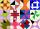Calculate the perimeter of isosceles triangle with arm length 26 cm and base length of 21 cm.
7. AnglesThe triangle is one outer angle 158°54' and one internal angle 148°. Calculate the other internal angles of a triangle.
8. Juice boxThe juice box has a volume of 200ml with its base is an isosceles triangle with sides a = 4,5cm and a height of 3,4cm. How tall is the box?
9. Triangle P2Can triangle have two right angles?
10. 3-bracket 2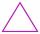May be the smallest angle in the triangle greater than 40°?
11. 7 triangle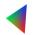The triangle area is 26.7 cm2. Determine the side length l if appropriate height hl = 45.3 cm.
12. 3-bracket 3Two angles in a triangle are 90° and 60°. Has triangle at least two equal sides?
13. Triangle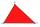For how many integer values of x can 16, 15 and x be the lengths of the sides of triangle?
14. Octagon from rectangleFrom tablecloth rectangular shape with dimensions of 4 dm and 8 dm we cuts down the corners in the shape of isosceles triangles. It thus formed an octagon with area 26 dm2. How many dm2 we cuts down?
15. MidpointsTriangle whose sides are midpoints of sides of triangle ABC has a perimeter 45. How long is perimeter of triangle ABC?
16. Area of RT 2Calculate the area of right triangle whose legs have a length 5.8 cm and 5.8 cm.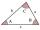The size of two internal angles of a triangle ABC are α=6/18π and β=7/18π. Calculate the size of the third angle.
18. QuizQ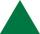An isosceles triangle has two sides of length 7 km and 39 km. How long is a third side?
19. Triangle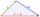Determine if it is possible to construct a triangle with sides 28 31 34 by calculation.
20. Triangle ABCIn a triangle ABC side b measure 10 cm less than the side a and side b is half of the side c. Calculate the length of sides if the circumference of the triangle is 42 cm.

Do you have an interesting mathematical example that you can't solve it? Enter it, and we can try to solve it.

To this e-mail address, we will reply solution; solved examples are also published here. Please enter e-mail correctly and check whether you don't have a full mailbox.